# C++语言程序化设计——第二次作业

## 五、教学过程

### 1、为什么要用函数（设计实验、教案说明）

（1）在面向过程化程序设计中，函数是模块划分的基本单位，是对处理问题过程的一种抽象。在面向对象的程序设计中，函数同样有着重要的作用，它是面相对象程序设计中对功能的抽象

（2）函数编写好后，可以被重复利用，使用时可以只关心函数的功能和使用方法而不必关心函数功能的具体实现。

（3）使用函数有利于代码重用，可以提高开发效率、增强程序的可靠性，也便于分工合作和修改维护。

double power(double x,int n){
double val=1.0;
while(n--)
val*=x;
return val;
}

int main(){
cout<<"5 to the power 2 is "<<power(5,2)<<endl;
return 0;
}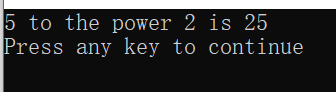### 2、为什么要用函数重载（设计实验教案说明）

（1）两个以上的函数，具有相同的函数名，但是形参的个数或者类型不同，编译器根据实参和形参的类型及个数的最佳匹配，自动确定调用哪一个函数，这就是函数的重载

（2）C++允许功能相近的函数在相同的作用于内以相同函数名定义，从而形成重载。方便使用，便于记忆。

（3）重载函数的形参必须不同：个数不同或者类型不同。

int sumOfSquare(int a,int b){
return a*a+b*b;
}

double sumOfSquare(double a,double b){
return a*a+b*b;
}

int main(){
int m,n;
cout<<"Enter two integer: ";
cin>>m>>n;
cout<<"Their sum of square: "<<sumOfSquare(m,n)<<endl;

double x,y;
cout<<"Enter two real number: ";
cin>>x>>y;
cout<<"Their sum of square: "<<sumOfSquare(x,y)<<endl;

return 0;
}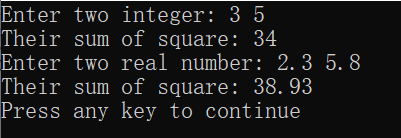### 3、什么是值传递（设计实验和教案说明其过程，分析其特性）

void swap(int a, int b){
int t=a;
a=b;
b=t;
}

int main(){
int x=5,y=10;
cout<<"x="<<x<<"     y="<<y<<endl;
swap(x,y);
cout<<"x="<<x<<"     y="<<y<<endl;
return 0;
}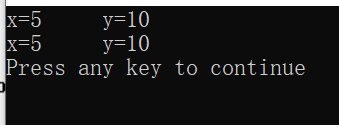### 4、什么是地址传递（设计实验和教案说明其过程，分析其特性）

（1）地址传递是将实参的地址传递给形参，从而可以通过改变形参使实参同步发生改变。

（2）在数据量大，或者需要在子函数中操作主函数空间的时候，通常使用地址传递。

void swap(int &a, int &b){
int t=a;
a=b;
b=t;
}

int main(){
int x=5,y=10;
cout<<"x="<<x<<"     y="<<y<<endl;
swap(x,y);
cout<<"x="<<x<<"     y="<<y<<endl;
return 0;
}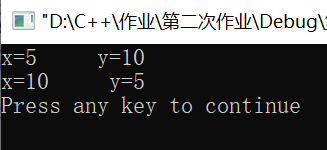### 5、设计实验和教案，分析如何编写递归函数

void move(char src,char dest)
{
cout<<src<<"-->"<<dest<<endl;
}

void hanoi(int n,char src,char medium,char dest)
{
if(n==1)
move(src,dest);//找出口
else
{
hanoi(n-1,src,dest,medium);
move(src,dest);
hanoi(n-1,medium,src,dest);//找模板
}
}

int main()
{
int m;
cout<<"Enter the number of diskes:";
cin>>m;
cout<<"the steps to moving "<<m<<"diskes:"<<endl;
hanoi(m,'A','B','C');
return 0;
}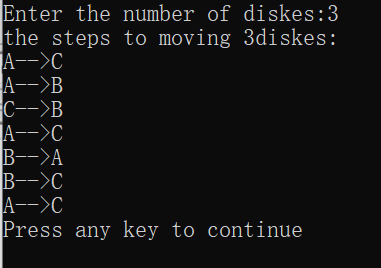posted on 2019-09-16 22:06  我喜欢宁宁宁  阅读(275)  评论(0编辑  收藏  举报